Refraction of Light

• Refraction of Light Happens in a Transparent medium when a light travels from one medium to another, refraction takes place.
• A ray of light bends as it moves from one medium to another Refraction is due to change in the speed of light as it enters from one transparent medium to another.
• The speed of light decreases as the beam of light travel from a rarer medium to the denser medium.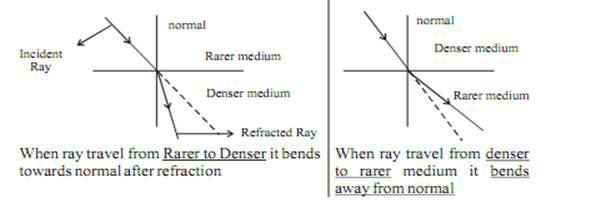Some Commonly observed phenomenon due to Refraction

• Rainbows.
• Light bending in a glass of water.
• Glasses.
• Camera lenses.
• Object dislocation in water.
• Binoculars.

Refraction through a Rectangular Glass Slab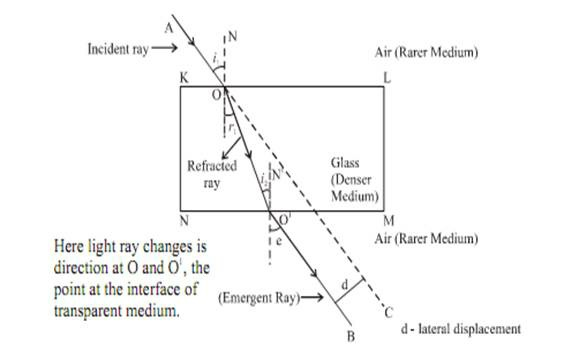When an incident ray of light AO passes from a rarer medium (air) to a denser medium (glass) at a point O interface on KL, it will bend towards the normal. At point O1, on interface NM the light ray entered from the denser medium(glass) to rarer medium (air) here the light ray will bend away from normal OO1 is a refracted ray OB is an emergent ray. If the incident ray is extended to C, we will observe that emergent ray O1B I parallel to the incident ray. The ray will slightly displaced laterally after refraction.

Note : When a ray of light is incident normally to the interface of two media it will go straight, without any deviation.

Laws of Refraction of Light

• The incident ray, the refracted ray and the normal to the interface of two transparent media at the point of incidence, all lie in the same plane.
• The ratio of the sine of the angle of incidence to the sine of the angle of refraction is a constant i.e.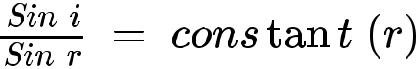For given colour and pair of media, this law is also known as Snell’s Law

Constant n is the refractive index for a given pair of the medium. It is the refractive index of the second medium with repect to the first medium.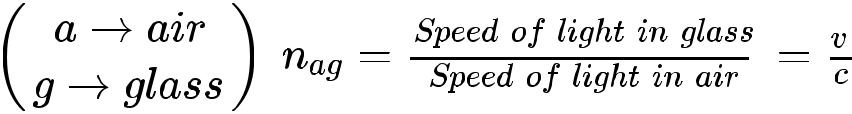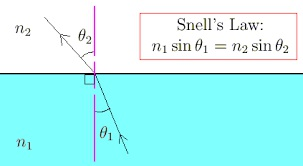Refractive Index

The refractive index of glass with respect to air is given by the ratio of the speed of light in air to the speed of light in glass.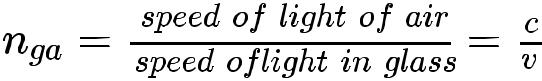Spherical Lens

A transparent material bound by two surfaces, of which one or both surfaces are spherical, forms a lens.

Convex lens

1. Bulging outwards
2. Bulging inwards.
3. Converging lens.
4. Diverging lens.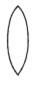Concave Lens

A lens bounded by two spherical surfaces, curved inwards is known asdouble concave lens (or simply concave lens). It is also known as a diverging lens because it diverges the light.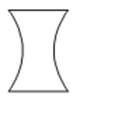Few Basic Terms Related to Spherical Lens

• Centre of curvature: A lens, either a convex lens or a concave lens is the combination of two spherical surfaces. Each of these surfaces from a part of the sphere. The centre of these two spheres are called centre of curvature represented by C1 and C2.
• Principal axis: An Imaginary straight line passing through the two centres of curvature
• Optical Centre: The central point of the lens is its optical centre (O). A ray of light, when passes through ‘O’ it remains undeviated i.e. it goes straight.
• Aperture: The effective diameter of the circular outline of a spherical lens.
• Focus of lens: Beam of light parallel to the principal axis, after refraction from

Convex lens, converge to the point on the principal axis, denoted by F, known as Principal focus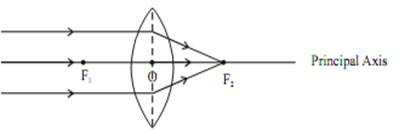Concave lens, appear to diverge from a point on the principal axis known as principal focus.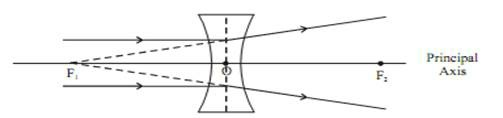The distance OF2 and OF1 is called as the focal length.

Tips for Draw among Ray Diagram

• After refraction, a ray parallel to principal axis will pass through F.
• A ray passes through F, after refraction will emerge parallel to principal axis

Lens formula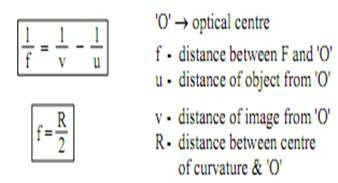Power of Lens

The degree of convergence or divergence of light ray achieved by a lenses known as the power of a lens. It is defined as the reciprocal of its focal length Represented by P.